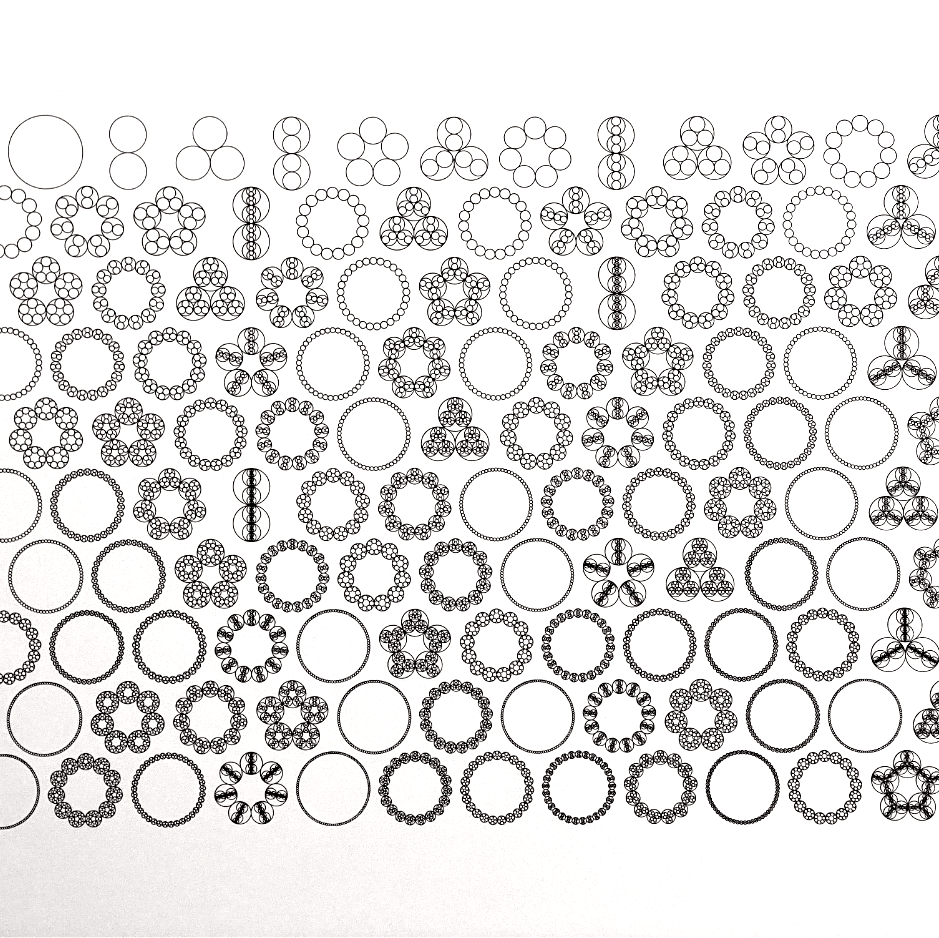# Sum-product withfew primes

Number theory

For a finite set of integers with few prime factors, improving the lower bound on its sum and product sets affirms the Erdös-Szemerédi conjecture.

## The sum-product problem for integers with few prime factors

Submitted (2023)

I. Shkredov, B. Hanson, M. Rudnev, D. Zhelezov

It was asked by E. Szemerédi if, for a finite set $A\subset \mathbb{Z}$, one can improve estimates for $\max{|A+A|,|A\cdot A|}$, under the constraint that all integers involved have a bounded number of prime factors -- that is, each $a\in A$ satisfies $\omega(a)\leq k$. In this paper, we answer Szemerédi's question in the affirmative by showing that this maximum is of order $|A|^{5/3−o(1)}$ provided $k\leq(\log|A|)^{1−\epsilon}$ for some $\epsilon>0$. In fact, this will follow from an estimate for additive energy which is best possible up to factors of size $|A|^{o(1)}$.

Submitted (2023)

I. Shkredov, B. Hanson, M. Rudnev, D. Zhelezov